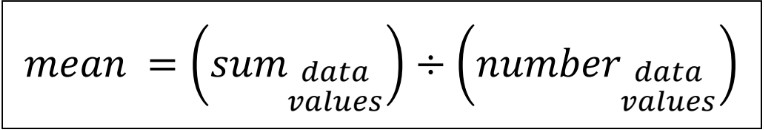# Introduction to mean, median, mode, and range#### Everything You Need in One Place

Homework problems? Exam preparation? Trying to grasp a concept or just brushing up the basics? Our extensive help & practice library have got you covered.#### Learn and Practice With Ease

Our proven video lessons ease you through problems quickly, and you get tonnes of friendly practice on questions that trip students up on tests and finals.#### Instant and Unlimited Help

Our personalized learning platform enables you to instantly find the exact walkthrough to your specific type of question. Activate unlimited help now!

0/6
##### Intros
###### Lessons
1. Introduction to Mean, Median, Mode and Range:
2. Analyzing data sets: what are the measures of central tendency?
3. Finding the range of a data set
4. Finding the median of a data set
5. Finding the mode of a data set
6. Finding the mean (average) of a data set
7. Review on mean, median, mode and range
0/17
##### Examples
###### Lessons
1. Range of a data set
Circle the biggest number, underline the smallest number, and calculate the range.
1. These are the scores of five students on a math quiz out of 20:
16, 17, 19, 16, 15
2. A group of friends recorded their scores in a video game:
75, 66, 81, 92, 84
2. Median of a data set
Find the median for the list of numbers
• Hint: the numbers must be in order from least to greatest
1. 1, 3, 5, 6, 8
2. 86, 47, 11, 52, 73, 62, 29
3. 18, 23, 25, 31, 36, 49
4. 100, 137, 96, 110
3. Mean (average) of a data set
Find the mean (average) of the numbers. Show your work.
1. 7, 4, 6, 5, 8
2. 17, 23, 16, 24
3. 8, 2, 3, 7, 5, 1, 9
4. Understanding the mean (average): word problem
The table below shows the quiz grades of five students.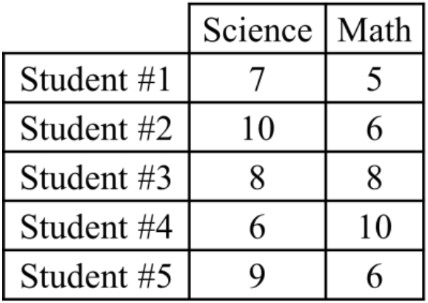1. What was the average grade for the science quiz?
2. What was the average grade for the math quiz?
3. On which quiz did the students do better on overall?
1. The teacher says that Student #3 did better on the Math quiz than on the Science quiz. Why is this true even though they have the same mark in both subjects?
2. For the students' English quiz, the five classmates had an average grade of 6. Find two possible sets of grades that would give an average grade of 6.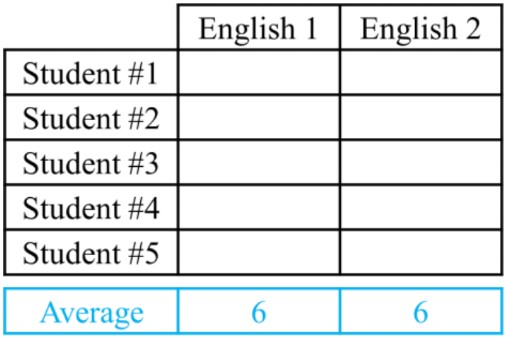5. Finding the mean, median, mode and range for a bar graph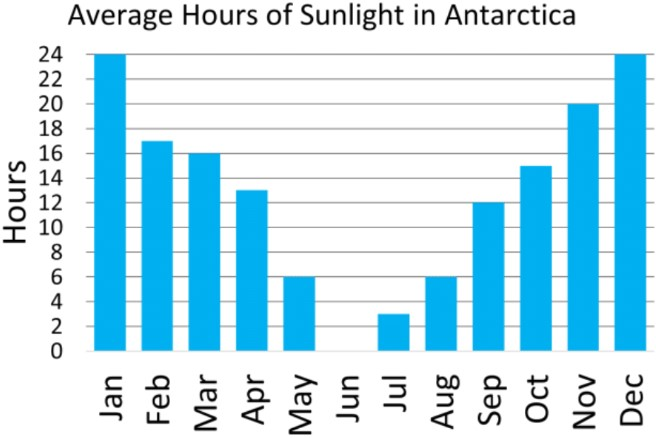1. Using the bar graph, fill in the numbers for the table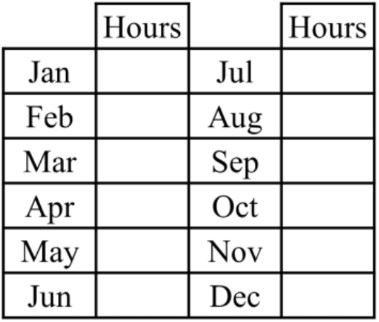2. Find the range of the data.
3. Find the mode of the data.
4. ind the median of the data.
5. Find the mean of the data.
###### Free to Join!
StudyPug is a learning help platform covering math and science from grade 4 all the way to second year university. Our video tutorials, unlimited practice problems, and step-by-step explanations provide you or your child with all the help you need to master concepts. On top of that, it's fun - with achievements, customizable avatars, and awards to keep you motivated.
• #### Easily See Your ProgressWe track the progress you've made on a topic so you know what you've done. From the course view you can easily see what topics have what and the progress you've made on them. Fill the rings to completely master that section or mouse over the icon to see more details.
• #### Make Use of Our Learning Aids###### Practice Accuracy

Get quick access to the topic you're currently learning.

See how well your practice sessions are going over time.

Stay on track with our daily recommendations.

• #### Earn Achievements as You LearnMake the most of your time as you use StudyPug to help you achieve your goals. Earn fun little badges the more you watch, practice, and use our service.
• #### Create and Customize Your AvatarPlay with our fun little avatar builder to create and customize your own avatar on StudyPug. Choose your face, eye colour, hair colour and style, and background. Unlock more options the more you use StudyPug.
###### Topic Notes

In this lesson, we will learn:

• How to calculate the four types of measures of central tendency for a data set: mean, median, mode, and range

Notes:

• Measures of central tendency refer to types of calculations done with data set numbers. A single number will describe the most common (central; middle) behaviors of the information you have collected.
• When doing experiments or surveys, the steps are to:
• (1) gather data
• (2) organize data
• (3) analyze data
• Analyzing data means that we want to make sense of all the information that we've gathered. What does the data tell us? What can we learn from it?

• There are four measures of central tendency: mean, median, mode and range.

• Range is the difference between the highest and lowest number
• It represents how far the data set is spread (how much it varies)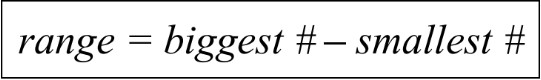• Median is the number listed in the middle of the data set (in order)
• A quick way to find the approximate center of the data
• To find the median, first list all numbers from least to greatest. Then count the number of data points (is there a total number that is odd or even?)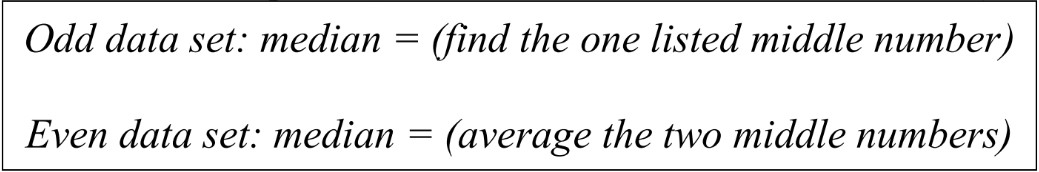• Mode is the most common data value; the one that shows up the most frequently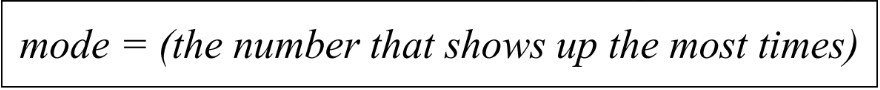• Mean (also called average) is the sum of all data points divided by the number of data points
• This is a better way to find the true middle of the data set; it spreads all the values across all data points, giving a better idea of what is happening overall